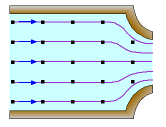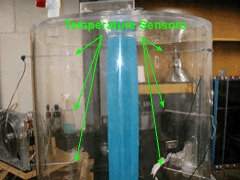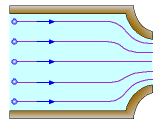Ch 3. Fluid Kinematics Multimedia Engineering Fluids FlowDescriptions Steady &Unsteady Streamlines,Streaklines Velocity &Acceleration IrrotationalFlow
 Chapter 1. Basics 2. Fluid Statics 3. Kinematics 4. Laws (Integral) 5. Laws (Diff.) 6. Modeling/Similitude 7. Inviscid 8. Viscous 9. External Flow 10. Open-Channel Appendix Basic Math Units Basic Equations Water/Air Tables Sections Search eBooks Dynamics Fluids Math Mechanics Statics Thermodynamics Author(s): Chean Chin Ngo Kurt Gramoll ©Kurt GramollFLUID MECHANICS - THEORY

To help analyze fluid flow, different viewpoints can be used, similar to using different coordinate systems. In this section, two different points of view for describing fluid flow will be discussed. They are the Eulerian and Lagrangian viewpoints.

Eulerian ViewpointEulerian ViewpointEulerian Example:
Temperature Measurements

The first approach to describe fluid flow is through the Eulerian point of view. The Eulerian viewpoint is implemented by selecting a given location in a flow field (x, y, z), and observe how the properties (e.g., velocity, pressure and temperature) change as the fluid passes through this particular point. As such, the properties at the fixed points generally are functions of time, such as

 V(x, y, z, t) Velocity T(x, y, z, t) Temperature ρ(x, y, z, t) Density p(x, y, z, t) Pressure

However, if the flow is steady, then the properties are no longer function of time. The Eulerian viewpoint is commonly used, and it is the preferred method in the study of fluid mechanics. Take the experimental setup as shown in the figure for example. Thermocouples (temperature sensors) are usually attached at fixed locations to measure the temperature as the fluid flows over the non-moving sensor location.

It should be noted that the position function, r, is not used in Eulerian viewpoint. This is a major difference from the Lagrangian viewpoint, which is used in particle mechanics (Dynamics and Statics).

When a fluid region is of interest, it is called a flow field and the velocity in that region would the velocity field. While it is easier to solve most fluid problem with the Eulerian viewpoint, it is also the harder one to mentally visualize since it deals with a region, and not a particular point or particle.

Lagrangian ViewpointLagrangian ViewpointLagrangian Example: Tracking of Whales

Another flow description is via the Lagrangian viewpoint in which a fluid particle is followed. This viewpoint is widely used in Dynamics and Statics, and easy to use for a single particle. As the fluid particle travels about the flow field, one needs to locate the particle and observe the change of properties. That is,

 r(t) Position T(xo, yo, zo, t) Temperature ρ(xo, yo, zo, t) Density p(xo, yo, zo, t) Pressure

where xo, yo, and zo represents a particular particle or object. One example of Lagrangian description is the tracking of whales (position only). In order to better understand the behavior and migration routes of the whales, they are commonly tagged with satellite-linked tags to register their locations, diving depths and durations.

In general, both Eulerian and Lagrangian viewpoints can be used in the study of fluid mechanics. The Lagrangian viewpoint, however, is seldom used since it is not practical to follow large quantities of fluid particles in order to obtain an accurate portrait of the actual flow fields. But the Lagrangian viewpoint is commonly used in dynamics, where the position, velocity or acceleration over time is important to describe in a single equation.

Practice Homework and Test problems now available in the 'Eng Fluids' mobile app
Includes over 250 problems with complete detailed solutions.
Available now at the Google Play Store and Apple App Store.